# Team:JNFLS China/Model

2016.igem.org/Team:JNFLS_China

We believe that an excellent model is supposed to be based on the experiment and be used on the experiment, which means to make assumption according to the data, and then use the principle we have learned(e.g. physics, chemistry, biology) to set up a model that can explain the phenomenon. By adjusting the parameter, we can achieve the best match between the final result and the model. If the result and the prediction of the model is always consistent when the condition is changing, we say that this model can be used. Hence we are able to use this model to predict the result by setting various parameters and observing the alter of simulations. The model has experienced. a complete process of "Found"-"Adjust"-"Test"-"Predict". Thus we start our journey of mathematical modeling.

# 1 Model of the process of the origin

Firstly, we set up a process model of the system without adding the miRNA. According to the principle of biology, the expression of DNA will follow the direction of the information flow of the central dogma when there’s no interference factor. (Fig. 1)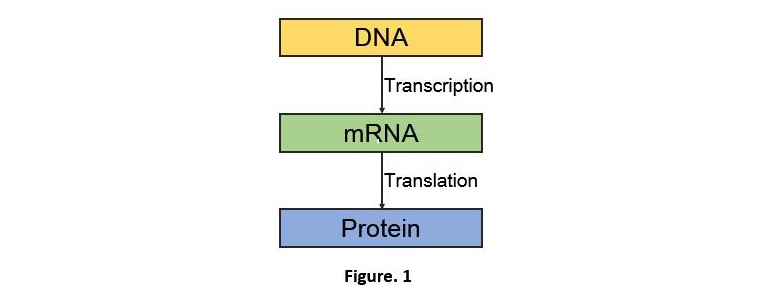Therefore, we get the reaction process 1 (Fig. 2).Ktc means the transcription rate,Ktl represents the translation rate.Kxdeg shows the degrading rate of matter x. Because the mass remains unchanged during the chemical reaction. We are able to get the ODE (ordinary differential equation) below (Fig. 3).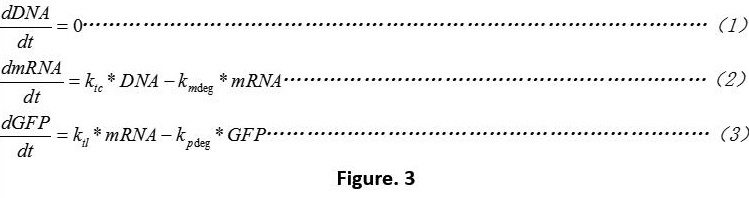The equation 1 means that we do not consider the influences of plasmid copy numbers.

After that we made simple setting of some basic parameter. (Table. 1) Use the software MATLAB R2016a to compile the script of the model (click here to download Script_1)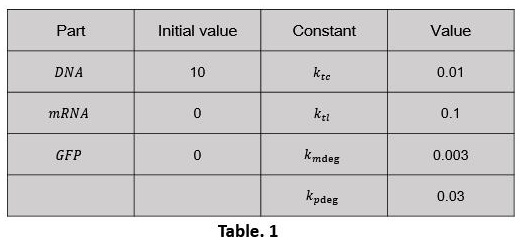The simulation results are shown below (Fig. 4):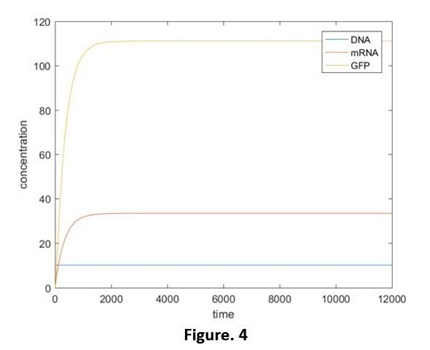Compared with the normal expression of green fluorescent protein (GFP), we found out in the experimental situation, the production of GFP won’t exist in the first 10 hours (Fig. 5). We have discussed this in figure mode(click here to check out our figure mode). We believed that in the lag phase, the concentration of the plasmid, the abilities of transcription and translation are very low. So it is not able to achieve to the steady state which the model predicts. Hence we should adjust the model.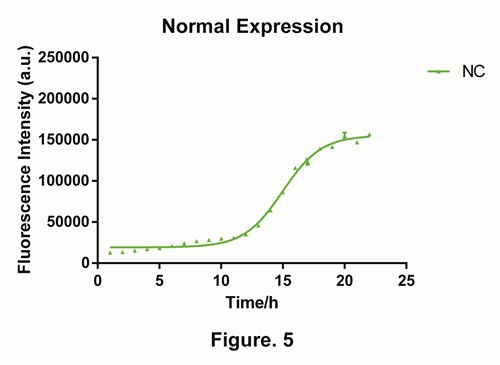We started with the express ability of bacteria itself. In our model, 2 parameters reflects the express ability: Ktc and Ktl To simples the analysis, the express ability are ascribed to Ktc. The assumption is: During the first 10 hours, the transcription speed is low; after the 11th hour, the speed recovers to the normal speed (Table. 2). In 0-6000 period, the value of Ktc is 0.001, and in the 6000-12000 period, the value will be 0.01. Use the software MATLAB R2016a to compile the script of the model (click here to download Script_2).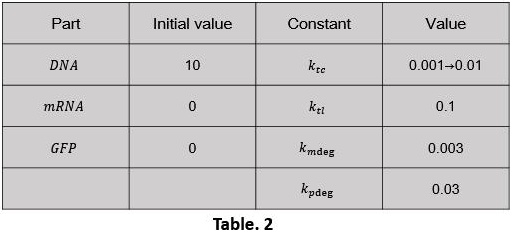The simulation results are shown below (Fig. 6):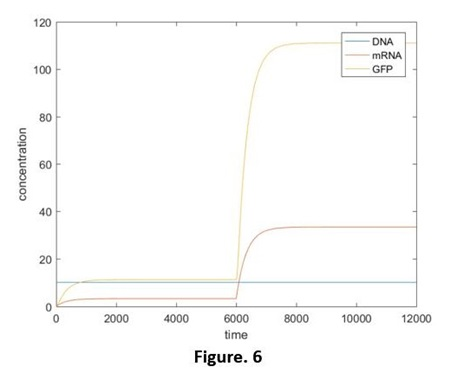The curve is relatively fit the experiment. Also there are differences on the slope of the simulation curve and the curve of our experiment. We believed that it is because the signals we set is changing very rapidly instead of changing gradually through time. To improve further, we believed that Ktc can also been seen as a function of some factors of growing. (We will improve further, later.)

# 2 Model of process of Device 1.0.

Secondly we started to consider the change of the system after miRNA is added. The key point is the modeling of the RNA-interaction. Through the process below, we simplified the RNA interaction: After adding RNA into the bacteria, it will contact with the binding site on the mRNA at a certain rate and form the RNA complex. However, at the same time it will also decompose to the miRNA and mRNA at a certain rate (The whole process is like a reversible reaction) (Fig. 7). Exclude those processes, both RNA complex and miRNA will be decomposed (or degraded) at a certain rate. The degrading rate of the RNA complex is a little faster. Considering most of the phages use ds RNA as their genetic material, the probability for bacteria to decompose miRNA is relatively big. Nevertheless, this issue has nothing to do with our main project, so we won’t discuss that. Thus we’ve got the process 2 (Fig. 8).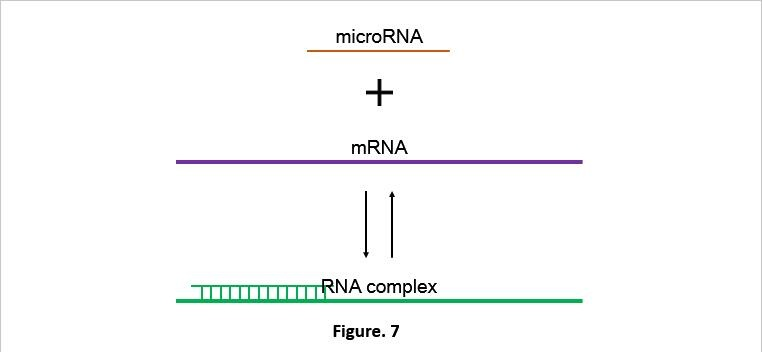K1 represents the connection rate of miRNA and mRNA.K2 means the degrading rate of RNA complex.Kxdeg represents the degrading rate of matter x.According to the law of conservation of mass and by connecting Figure. 2. We are able to get the ODE below (Fig. 9):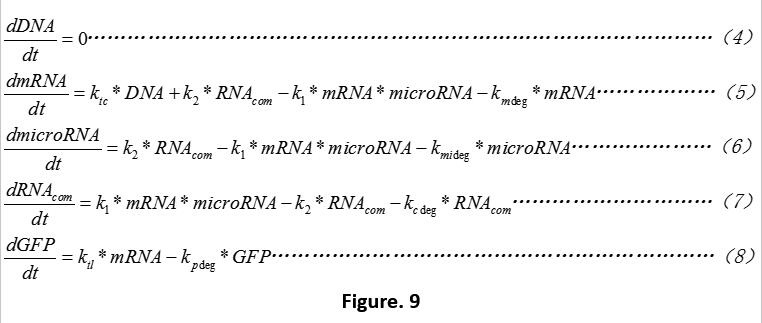Because we are not able to get any appropriate setting value for the original concentration of miRNA from any experimental data, consider the growing situation of bacteria may lead to the missing of the "window period". (Know more by reading the “failure mode”) So we explain the process of this based on the Script_1 without considering the dilution effect.

After that we made simple setting of some basic parameter (Table. 3). Use the software MATLAB R2016a to compile the script of the model (click here to download Script_3).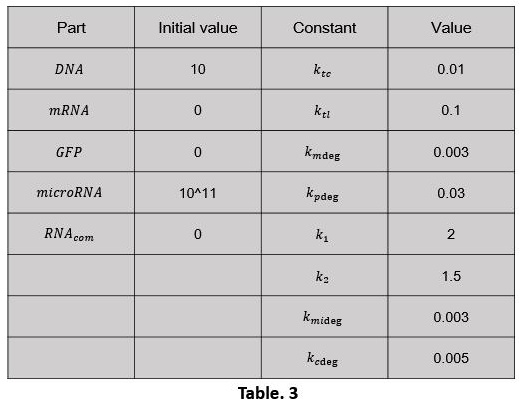The simulation results are shown below (Fig. 10, 11):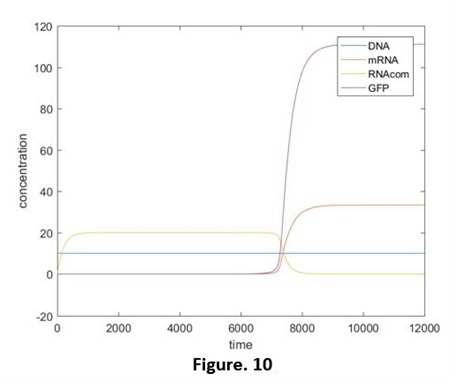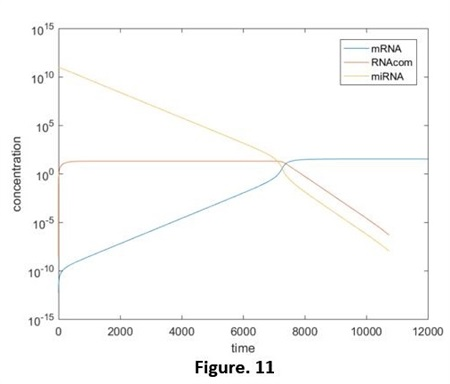From these 2 figures we got that: without the extra production of miRNA, the concentration of it is continuing decreasing and the highest rate appeared at the very beginning. ( y-axis of Fig. 11 is the logarithmic coordinates.) Compared the adding of miRNA and the independent miRNA (It can be seen as the normal expression of system 1.0. Fig. 12, did not show the relative script), we are able to see the significant restrain of expression, as the degrading of miRNA, the quantity of green fluorescent protein start to incline.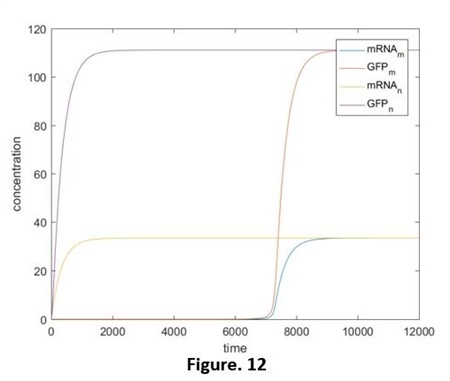At the beginning, the concentration of miRNA is much higher than that of mRNA, in the balance of “Connecting- Degrading”, the direction of producing RNAcom is in a dominant position. That is to say, almost all the mRNA is in the form of RNAcom. Until t=7000 or so, because of the degrading effect, the concentration of miRNA reduce to a threshold value and then it continuing falls, the balance of the reaction start to move to the decomposing direction. mRNA exists as an independent form from now on. Therefore, the translation process is opened and the green fluorescent protein starts to express.

On the foundation of the models above, we consider the expression ability of the bacteria and adjust the reacting rate. The other parameters states the same as Fig. 2. Use the software MATLAB R2016a to compile the script of the model (click here to download Script_4).

The simulation results are shown below (Fig. 13, 14):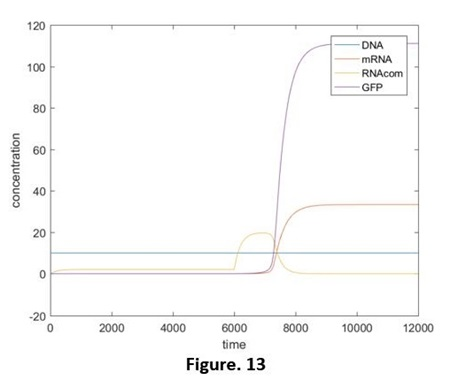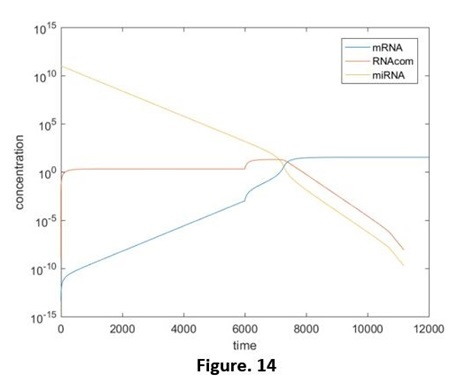Compared those 4 diagrams, the RNAcom that be produced reduced a little after considering the expression ability, this is restrained by the concentration of mRNA. However, after that, the transcription rate will recover before the concentration of miRNA declines to the effective concentration. Hence, by comparing adding the miRNA and adding the independent miRNA, we still are able to figure out the reduction of the effects, and that is the same as our results in the experiments. (Fig. 15, 16, without the script) But if the speed of the transcription rate recover after the concentration of the miRNA reduces to the effective concentration (e.g. Ktc rises again at the time t=8000). The effective reduction will be not able to be observed (Fig. 17, without the relative script), no matter whether we add the target RNA, the results will be the same. That promotes us that our designing has limitations: if the concentration is lower than a threshold value, the difference will not be detected.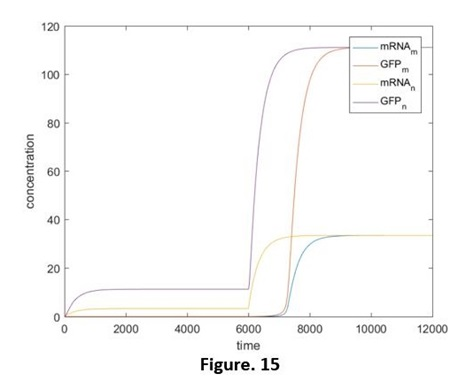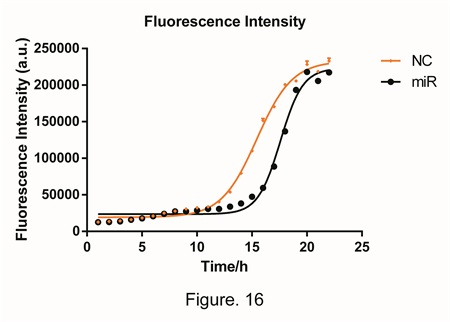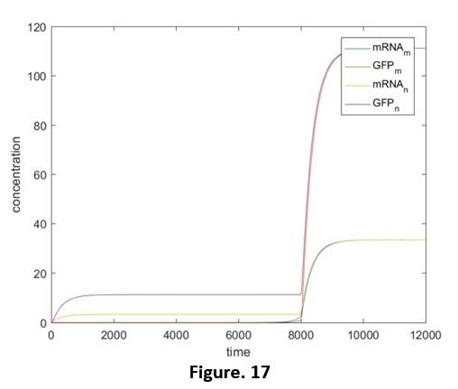aa

# 3 Use △GFP to reflect the concentration of miRNA

By analyze the figure 16., we minus the concentration when the target miRNA was added by the concentration when an irrelevant miRNA was added, and hence we got the process of △Fluorescent intensity (Fig. 18). The trend of the data is similar to a bell curve and the peak value occurs at the 15th. We believe that the difference in fluorescent intensity (or we can say the quantity of GFP), is highly relevant to the concentration of miRNA. In theory, both the peak width and the peak height are able to reveal the quantity of miRNA. However, because the detection of the width requires over 10 hours. We decide to use the peak height, which is also the △GFPmax, to calculate further.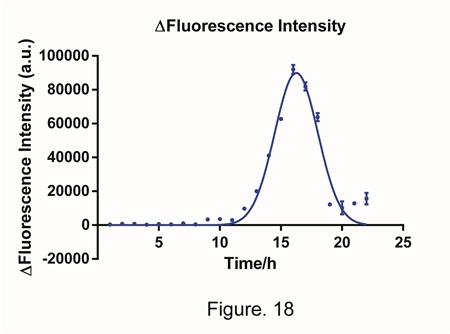Through the predicting of the model, we have gained a series of data of relationship between △GFPmax and the concentration of miRNA .We fit a curve, and by the fitting is good (Fig. 19, focus that the x-axis is exponential coordinates). When the experimental data and the math modeling is completely fit with each other, the model can be used to predict. Therefore, we can predict the concentration of the miRNA in the sample by measuring the △GFPmax.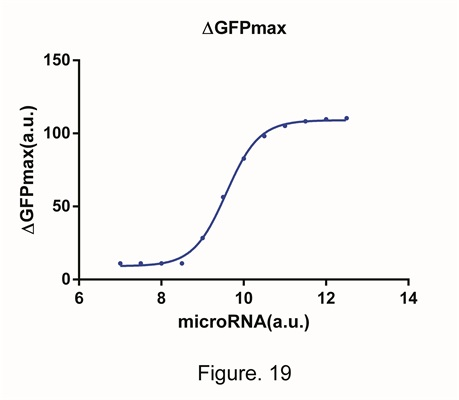From Fig.19, we can also gain the information of the scope of application. In the figure, the region that the curve shows best is 10^9~10^10. Under the 10^9, the concentration of miRNA will soon decline under the effective scope and cannot be detected. When the concentration is higher than 10^10, the expression of GFP is restrained completely and reach 0. However the negative value is impossible. At that time the system reached its limit and no matter how high the concentration of miRNA is, the system cannot reflect that.

# 4 CONCLUSION

We started by the most fundamental rule-the central rule. Then we set up the model of the whole reaction step by step. We made the appropriate assumption of the experiment through the data, adjusted the parameter to let it fit the model, and achieve that finally. From the model, we found the variant that can reflect the alter of concentration of miRNA and describe it successfully, and explain the effective scope of it.

Because of the limit of the time, we can only build the model of system 1.0 completely. The model of system 2.0 will be more complex. It might have to do with the concentration of repress protein and the jump range of it and more various factors. We wished that in the future, there will be new team to improve the model and give better fitting methods.